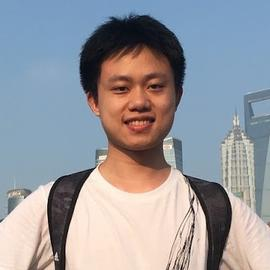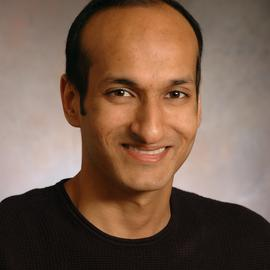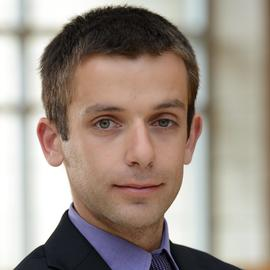# Dynamic Regret Minimization for Control of Non-stationary Linear Dynamical Systems

### Abstract

We consider the problem of controlling a Linear Quadratic Regulator (LQR) system over a finite horizon $T$ with fixed and known cost matrices $Q,R$, but unknown and non-stationary dynamics $A_t, B_t$. The sequence of dynamics matrices can be arbitrary, but with a total variation, $V_T$, assumed to be $o(T)$ and unknown to the controller. Under the assumption that a sequence of stabilizing, but potentially sub-optimal controllers is available for all $t$, we present an algorithm that achieves the optimal dynamic regret of $\tilde {\cal O}\left(V_T^{2/5}T^{3/5}\right)$. With piece-wise constant dynamics, our algorithm achieves the optimal regret of $\tilde {\cal O}(\sqrt{ST})$ where $S$ is the number of switches. The crux of our algorithm is an adaptive non-stationarity detection strategy, which builds on an approach recently developed for contextual Multi-armed Bandit problems. We also argue that non-adaptive forgetting (e.g., restarting or using sliding window learning with a static window size) may not be regret optimal for the LQR problem, even when the window size is optimally tuned with the knowledge of $V_T$. The main technical challenge in the analysis of our algorithm is to prove that the ordinary least squares (OLS) estimator has a small bias when the parameter to be estimated is non-stationary. Our analysis also highlights that the key motif driving the regret is that the LQR problem is in spirit a bandit problem with linear feedback and locally quadratic cost. This motif is more universal than the LQR problem itself, and therefore we believe our results should find wider application.

Type
Publication
Accepted to SIGMETRICS 2022##### Yuwei Luo
###### MS Student (2019-2020)

Yuwei Luo received his M.S. degree in Statistics at University of Chicago in March 2020. Prior to graduate school, he received B.S.degree in Mathematics at University of Science and Technology of China (USTC) in June 2018. His research interests include reinforcement learning, control, optimization and network analysis. Yuwei is continuing his education as a PhD student at Stanford University.##### Varun Gupta
###### Associate Professor of Operations Management

Varun Gupta is an Associate Professor of Operations Management.